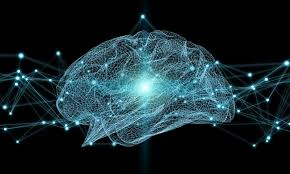### Mathematical Neuroscience - M1 - 8EC

Content
Mathematics is becoming an increasingly important topic in the neurosciences. Contemporary neuroscience research adopts a variety of approaches including heuristic, biophysical, computational and data-driven modelling. As neuroscience models become more complex and ambitious, mathematical analysis is expected to contribute in pivotal ways: (i) establishing rigorous foundations for the models conceived in the field, (ii) proposing analytical and numerical strategies to bridge spatio-temporal scales, (iii) identifying key features and common model behaviours, and, ultimately, (iv) providing a common language to facilitate cross-contamination between applied and theoretical neuroscience.

The aim of this course is twofold: explaining how mathematical models of cerebral activity can be derived from first principles, and introducing mathematical methods for their analysis, with the view of reproducing experimental data and predicting spatio-temporal cortical signals. As neuroscience models are naturally framed as dynamical systems, we will use methods from bifurcation theory, geometrical singular perturbation theory, and pattern formation to analyse models at various scales:

• At single cell level, we will review biophysical (Hodgkin--Huxley type) models and their simplifications, discussing mathematical mechanisms explaining spiking, bursting, mixed-mode oscillations, and other temporal signals.
• At network level, we will examine how synchronisation arises through neuronal coupling. We will also introduce and analyse neural mass and other mean-field models. Emphasis will be given to the derivation of such models from the single-cell ones, highlighting the assumptions and challenges of this approach.
• At cortical level, we will study the formation of waves and coherent structures in nonlocal, nonlinear, spatially-extended neural field models.

Prerequisites
The course will assume previous knowledge of nonlinear ODEs, in particular phase plane analysis. Tools from bifurcation analysis will be refreshed in this course, but experience with bifurcation theory (saddle-node, pitchfork, and Hopf and homoclinic bifurcations) will help. A familiarity with numerical methods (time stepping, root finding) and coding in Matlab, Python, or other programming languages is also required.

Learning goals
After this course the student is able to:

• derive mathematical models of the brain at various spatial and temporal scales;
• select appropriate biophysical models to describe neuroscience phenomena;
• explore dynamical-systems behaviour using a variety of mathematical techniques including: bifurcation analysis for ODEs and spatially-extended systems, numerical methods, multiple-scales analysis;
• critically interpret model analysis, in relation to experimental data.

Course delivery
The course will be taught using a combination of modelling lectures, analytical sessions, and numerical demonstrations. Each week we will have a lecture followed by a 1-hour tutorial.

In Spring 2023, live lectures will be delivered on an online platform, will be recorded, and made available afterwards. The tutorial will not be recorded.

Lecturers

* Daniele Avitabile (Vrije Universiteit Amsterdam) d.avitabile@vu.nl
* Hil Meijer (University of Twente) h.g.e.meijer@utwente.nl
Correspondence should be addressed jointly to the lecturers.